# On the distribution of the increments of the logarithms of H + L days (“I haven’t picked up checkers for a long time”)I did this research under the influence of a heated discussion on the forum about the distribution of “tails” of increments of logarithms of prices, which seemed to arise out of nowhere: how correct are the confidence intervals for estimates of linear regression parameters in the alpha-beta model?

Indeed, these estimates in the classical case are based on the central limit theorem for statistics of estimates of linear regression parameters. However, as I wrote on the smartlab, the necessary condition of which is the growth rate of the variance of the sum of terms as O (N), N is the number of terms, and for fast convergence in the central region, the finiteness of the absolute third moment of any term is also required (if we talk about the convergence on the whole line, including “large deviations”, then the finiteness of all moments of individual terms is also required). However, these conditions are not met for the part of the Pareto and Student distributions with a polynomial rate of decay of the “tails” and, therefore, for a “good” approximation of the sum of such terms by the normal law, a very large number of tests are required, which, as a rule, in an alpha-beta model built on daily No data. This means that traditional methods of constructing confidence intervals for estimating the parameters of this model “do not work.”

In fact, the discussion later boiled down to the question: are the “tails” of daily increments of logarithms of prices distributed according to the Pareto distribution, that is, with a polynomial decrease O (x-but) or we are dealing with exponential decay of the form О (eaxxb), a> 0, b-any.

The first result is based on the selection of separate “tails” and their approximation by the Pareto distribution. In this case, the central region is ignored, since it is impossible to approximate the entire distribution of the increments of logarithms of prices by the Pareto distribution. And what speaks in favor of the second hypothesis?

In my video from the 21st to 32nd minutes, I present the reasoning on the basis of which, for the increments of the logarithms H + L (ht) days will be distributed

with the rate of decrease of the “tails” O (eaxx-1/2), K0-MacDonald function.

It also shows the graphical “similarity” of this distribution to the distribution of the increments of the logarithms of the H + L futures on the RTS index in 2005-2016 with the “crisis” period thrown out from September 17, 2008 to February 28, 2009. However, no results of statistical studies are provided. Let’s fill this gap using the example of SPY on data from 01/29/1993 to 05/24/2021.

Before proceeding to the results, let us explain what the increments of the logarithms of H + L are. In the early 2000s, I found out that for RAO UES and Gazprom they have a correlation of more than 0.99 with the increments in the logarithms of the weighted average daily prices. That is, we are dealing with a number of increments in the logarithms of the weighted average daily prices, which, IMHO, more accurately reflect the picture of daily sentiment than the closing prices, that is, prices at a particular moment of the day. Why SPY, and not S & P500, whose history is much longer? The point is that the index data does not take into account intraday gaps due to the equality open today = close yesterday. And if there is a gap, we get that in H or L there may be prices that were not at all in the auction.

Let’s take the whole series of daily increments of logarithms and approximate them with the indicated distribution. For densities, we get the following picture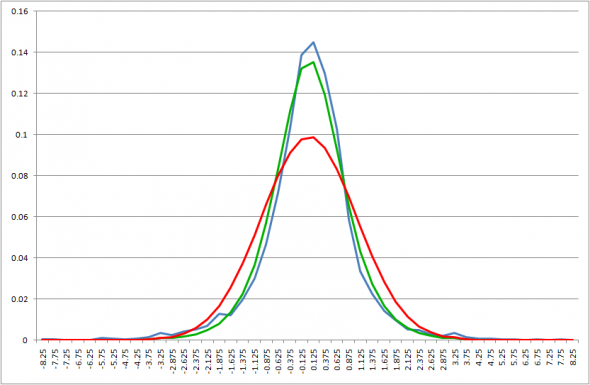Hereinafter, on the graphs, the sample density of distribution of the increments of the logarithms of prices normalized by the standard deviation is shown in blue, green is the closest density expressed in the above formula (we denote it by Kabout by analogy with the McDonald function), and in red – the density of the normal distribution with the same mean as for the normalized increments of logarithms of prices and variance 1 (recall that the variance of the normalized series of increments of logarithms is also equal to 1).

Despite the visual “closeness” of the green and blue graphs, the value of Kolmogorov’s statistics rejects the hypothesis of coincidence of distributions with a probability of a type I error of 0.05 (see the summary table below: the critical value of statistics for a probability of a type I error of 0.05 – 1.36). So, throughout history, it was not possible to obtain an approximation by the indicated distribution. However, in the aforementioned video, I also threw out the sample values ​​for the crisis period from September 17, 2008 to February 28, 2009, having specially stipulated that during this period, most likely, this distribution is far from reality.

And let’s, by analogy with the factor analysis from the video, divide the entire period into clusters of “volatility” and see what happens separately on each of the clusters.

Under the current “volatility”, as in the case of factor analysis, we mean the maximum of two values:

– sigma estimate from the mentioned distribution by increments of logarithms of H + L for the last 50 days (less is impossible due to the estimation error), i.e., under the assumption that the parameters of this distribution were constant during these 50 days;

– Standard deviation of the same increments of logarithms for the last 10 days.

Why is that? The second estimate is very inaccurate, but it allows you to quickly react to the increase in volatility. While the former will “see” the real growth only after about 25 trading days due to the shift in the “window” of the calculation. The main mistake of this calculation is that a one-day spike in the logarithm increment can be taken as a new cluster of higher volatility within 9 days, until this one-day spike leaves the calculation. But from the point of view of risks, this mistake is less critical than the mistake of missing a real increase in volatility, which is less likely with this approach.

And, by the way, for sufficiently widely spaced time intervals, the sigma estimates can be very different, which indicates that this parameter is not stationary. However, there are no large “steps” (more than 25% of the previous value) in none of its sample series, spaced by 25 points. This indicates the absence of large “gaps” (at times) in this value and its relatively “smooth” variability. This means that the same “tails” in the original sequence are either serial and appear as a result of a gradual increase in “volatility”, or are isolated and extremely rare (“black swans”).

Here are the clusters we got.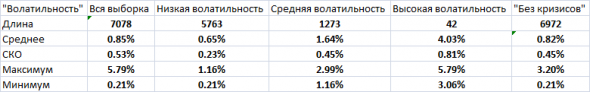About the column “No crises” a little later.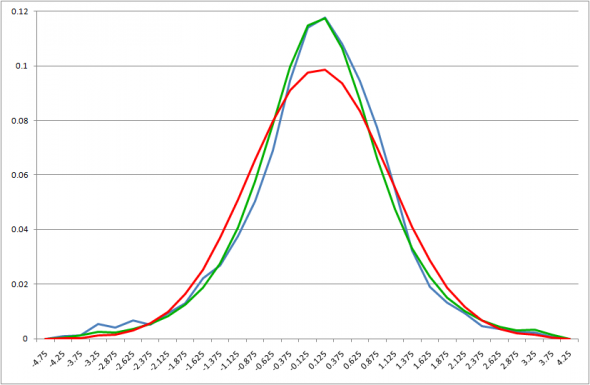And we immediately get by the Kolmogorov criterion that the hypothesis about the coincidence of distributions for our distribution cannot be rejected. Although the hypothesis of normality is rejected by this criterion.

Even better according to the same criterion is obtained for “average” volatility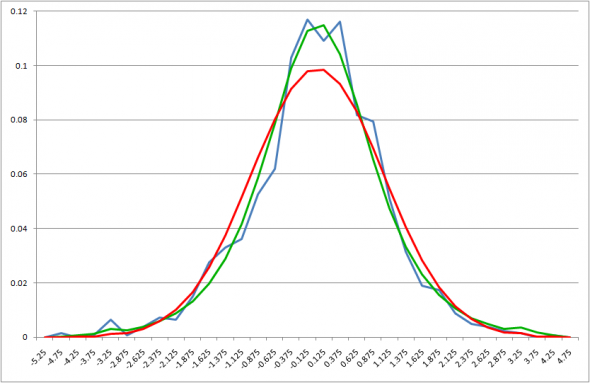The normal distribution is still out of the game.

And for “high” “volatility” the Kolmogorov criterion gives us a match with our distribution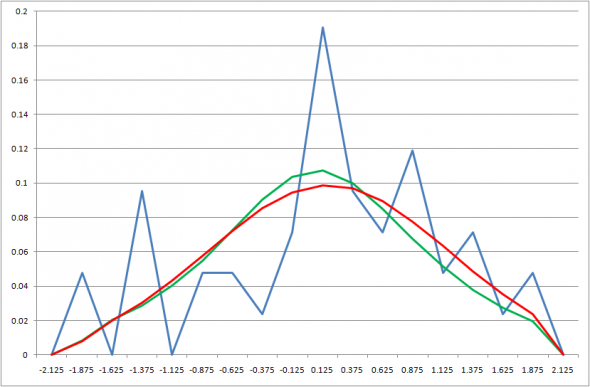And even a coincidence with a normal one is obtained by this criterion. But we must take into account that after normalization, we got a very “pretentious” distribution, lying in the range [-2,2] and a total of 42 points. By the way, the “pretentiousness” of this distribution indirectly indicates that the “super-heavy tails” are a product of the “volatility” in our definition.

We also note that of the last 42 points, 40 fell on the periods 17.09.2008-15.12.2008 and 24.02.2020-10.04.2020, that is, the “acute phases of crises”. Therefore, the last step is to discard these periods from the data and see what happened “Without crises”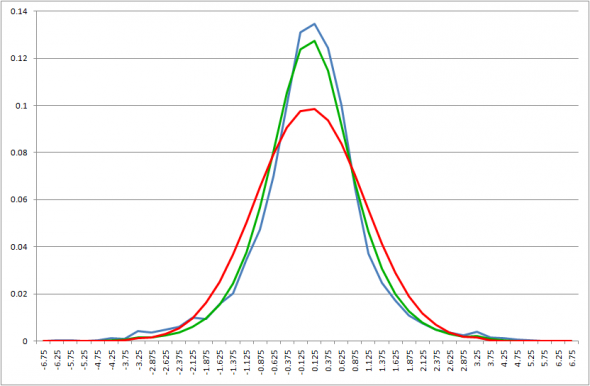And even for this sample, Kolmogorov’s test gives us a coincidence with the distribution Kabout, albeit “thin”. Well, the normal is still “out of the game”. A summary of the values ​​of the Kolmogorov statistics is given in the following table.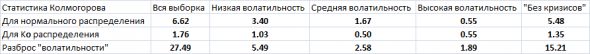We also note the explicit dependence of the values ​​of the Kolmogorov statistics on the range of fluctuations of our “volatility” for the selected period, which suggests that for periods with a smaller range, most likely, the approximation of the sample distribution by the distribution Kabout will be even better.

Thus, the hypothesis put forward in the video that the given distribution well “explains” the one-dimensional distribution of the increments of the logarithms of the H + L prices for daily periods outside the periods of “acute phases of crises” was fully confirmed on SPY.##### Fidenge Pecold

My profession is a journalist, but my hobby for 8 years has been studying Forex investing and trading. During this time, I managed to gain extensive experience in investing and trading cryptocurrencies and double my capital in the Forex market. To be the author of this magazine, the site owners invited me to participate in one of the 2020 trading webinars, and I will try to reveal the most relevant crypto market news for you.